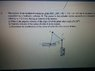# Mechanical engineering mechanics question: Motions of a triangular plate

• sophiebeth100
In summary, the conversation involves solving a physics problem involving a rotating triangle. The participants discuss the use of the formula w=v/r and the importance of using the tangential velocity when applying it. They also discuss the relationship between the angular velocity and the angle of rotation, and the importance of taking into account the movement of point C in solving the problem. The final solution involves calculating the time using the distance and speed, and then calculating the angular velocity using the formula w=dθ/dt.

Shown in picture

## Homework Equations

So far just used w=v/r

## The Attempt at a Solution

[/B]
Could only do part a.

W=v/r
=0.3/0.2

For part b I would assume the velocity is zero? But I don't think that's correct. Any help with part b and c would be great, and is part a correct? Thank you :)

#### Attachments

•IMG_20160504_111321.jpg
64.2 KB · Views: 414
Hi beth,funny to see 'Shown in picture' for the problem statement, right next to the picture. It doesn't say which half of the picture constitutes the problemBvU said:
Hi beth,funny to see 'Shown in picture' for the problem statement, right next to the picture. It doesn't say which half of the picture constitutes the problemCould you be more specific in regards to my answer for a? Did I use the right formula? Is there a different formula I should be using?

I asked first ! Where are your v and r and what is your axis of rotation ? Don't you become suspicious if the answer doesn't seem to depend on ##\beta## ?

BvU said:
I asked first ! Where are your v and r and what is your axis of rotation ? Don't you become suspicious if the answer doesn't seem to depend on ##\beta## ?

I used the v for the hydraulic cylinder, 0.3, and the radius 0.2, as the edge of the triangle is.

I think I have found my mistake - I had assumed B was the centre of the circular motion but I now see that B can move and this is wrong. Since there is no fixed point on the triangle, I don't know where to go from here

Oh boy, back to the drawing board.

There was something else I wanted to bring up: your equation comes from ##\ \ \vec v = \vec \omega \times \vec r\ \ ##, a vector product. You familiar with that ? In other words, if you want to write ##\omega = v/r## you can't just take any ##v##: you need the tangential velocity (the component perpendicular to ##\vec r##).

With that, do you now see an opening to get going with this exercise ?

BvU said:
Oh boy, back to the drawing board.

There was something else I wanted to bring up: your equation comes from ##\ \ \vec v = \vec \omega \times \vec r\ \ ##, a vector product. You familiar with that ? In other words, if you want to write ##\omega = v/r## you can't just take any ##v##: you need the tangential velocity (the component perpendicular to ##\vec r##).

With that, do you now see an opening to get going with this exercise ?

I see. So when beta=30, the side CB is vertical. However, since CB=r, wouldn't the perpendicular velocity=0, since the machine only operates up and down?

Yes, they made a deceiving drawing. On purpose ?
Even if CB is vertical, the thing is rotating when A moves upwards: B moves to the right. So ##\omega \ne 0##.

Can you see a relation between ##\omega## of CD and ##\omega## of BA ?typo: between ##\omega## of CB and ##\omega## of BA

Last edited:
sophiebeth100 said:
However, since CB=r, wouldn't the perpendicular velocity=0, since the machine only operates up and down?
Not because the machine only operates up and down. But because B only moves horizontally. So if you answer b) first you do have an answer for a) -- but you have to take into account that C also moves !
But perhaps the composer of the exercise wasn't that devious after all and gave you part a) first for a reason...

BvU said:
Yes, they made a deceiving drawing. On purpose ?
Even if CB is vertical, the thing is rotating when A moves upwards: B moves to the right. So ##\omega \ne 0##.

Can you see a relation between ##\omega## of CD and ##\omega## of BA ?Do you mean of CB and BA? But hmm are they the same?

Very good. Next step: any relation with ##\beta## ?

BvU said:
Very good. Next step: any relation with ##\beta## ?

I really can't find a relationship between those two things

What can you say about ##\beta## if the triangle does not rotate (only translates) ?

BvU said:
What can you say about ##\beta## if the triangle does not rotate (only translates) ?

The value stays the same?

Yes. So what is ##{d\over dt} \beta## when it rotates ?

BvU said:
Yes. So what is ##{d\over dt} \beta## when it rotates ?

Is that the change in β related to time? Where does time come into it?

You want ##\omega## at the moment that ##\beta = {\pi/6}##. A is moving, remember ?
Do you know the definition of ##\omega## ? It is the time derivative of an angle. What angle in your exercise ?

BvU said:
You want ##\omega## at the moment that ##\beta = {\pi/6}##. A is moving, remember ?
Do you know the definition of ##\omega## ? It is the time derivative of an angle. What angle in your exercise ?

Ah I see, so ω=dθ/dt

So to calculate the time, use time = distance / speed.
Not 100% sure on this, but is the distance 0.1m? Since it is half the height of one side of the triangle?
If that's right, time = 0.1/0.3 = 0.33s

ω=dθ/dt
ω=(π/6)/0.33